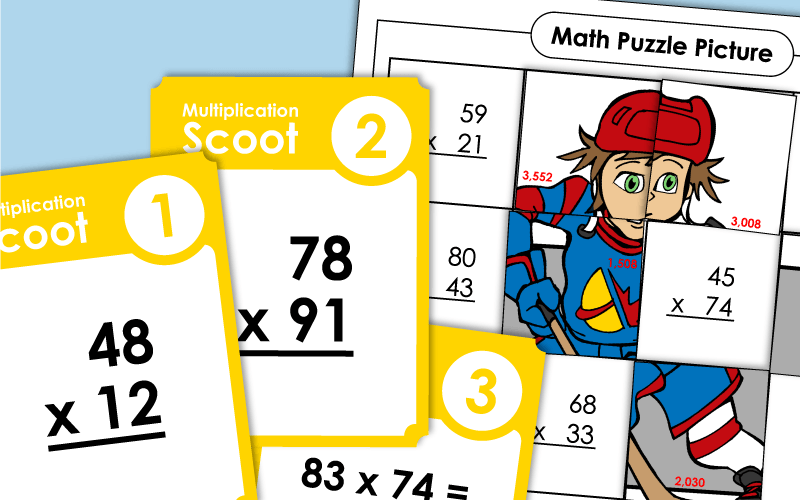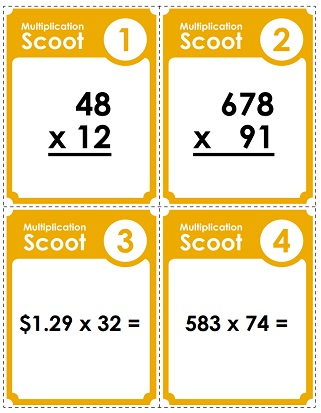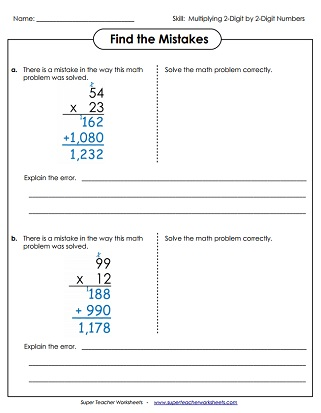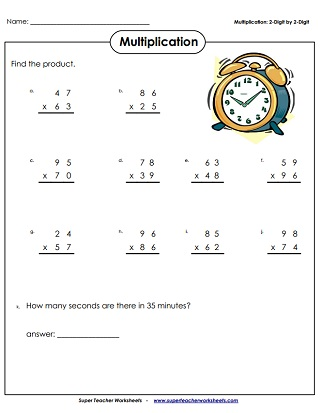# Multiplication (2-Digits Times 2-Digits)

The worksheets below require students to multiply 2-digit numbers by 2-digit numbers. Includes vertical and horizontal problems, as well as math riddles, task cards, a picture puzzle, a Scoot game, and word problems.## 2-Digit Times 2-DigitWorksheets

Practice 2-digit by 2-digit vertical multiplication problems. (example: 85 x 62)
This variety worksheet has an array of 2-digit by 2-digit math problems, including two word problems, an input/output table, and vertical and horizontal equations.
At the top of this worksheet, students are shown a dozen shapes with two-digit numbers in them. They multiply similar shapes. For example: Find the product of the numbers in the hexagons.
When you examine these two completed multiplication problems, you'll observe that each one has an error. Find the error and explain it in words. The re-solve the incorrect problem.
Begin by using the cipher key to decode the secret symbols. Then multiply the factors together to calculate the products.
A practice page with a cute dog theme; 2-digit numbers by 2-digit numbers (example: 46 x 34)
Graph Paper Math Drills; 2-digits times 2-digits (example: 18 x 22)
Word problems for double-digit multiplication practice (example: 56 x 91)
Find the products of the 2-digit factors. All problems are written horizontally. Students rewrite each problem vertically and solve. (example: 76 x 23)
Solve the 2 digit by 2 digit multiplication problems. Then attach the puzzle pieces in the correct positions to reveal an ice hockey picture.

## Multi-Digit MultiplicationWorksheet Generator

Multiplication Worksheet Generator

Here's a tool that you can use to make your own customized PDF math worksheets. You can select the number of digits in both factors. You can also toggle between horizontal and vertical problems.

In this classroom game, students solve a series of math problems on task cards. The cards have 2-digit by two-digit multiplication problems on them.
These printable task cards have thirty 2-digit by 2-digit multiplication problems.

## Lattice Multiplication

At the top of the worksheet is a box with an explanation of how to do lattice multiplication. Then students can try to solve the eight sample problems using a lattice grid.
Here are six two-by-two lattice grids. The teacher or students can input any numbers they'd like.
Multiplication Worksheets

This index page can direct you to any type of multi-digit multiplication worksheets on our website. Includes multiplying by 1, 2, and 3-digit numbers. Also have money, decimal, and fraction multiplication.

## Sample Worksheet ImagesMy Account
Site Information